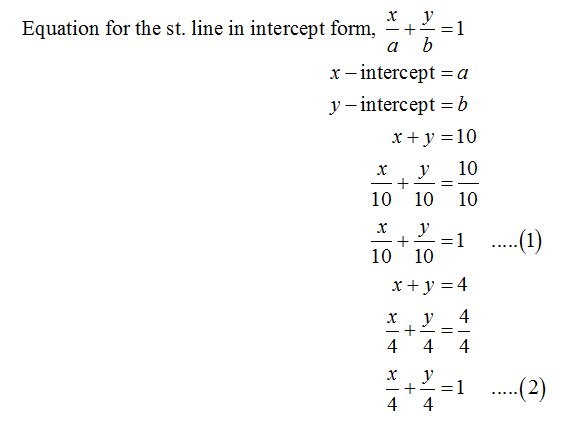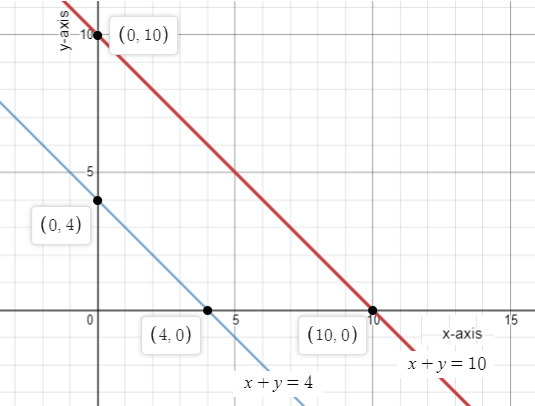Question
1 views

Solve the system of equations by graphing.

x+y=10

x+y=4

check_circle

Step 1

First of all, consider the equation for the straight line in the intercept form.

Rearrange the given 2 equations in the equation for the straight line in the intercept form.Step 2

Plot the graphs for equation (1) and (2).

The x-intercept and y-intercept for the equation (1) are 10 and the x-intercept and y-intercept for the equation (2) are 4....

### Want to see the full answer?

See Solution

#### Want to see this answer and more?

Solutions are written by subject experts who are available 24/7. Questions are typically answered within 1 hour.*

See Solution
*Response times may vary by subject and question.
Tagged in

### Algebra